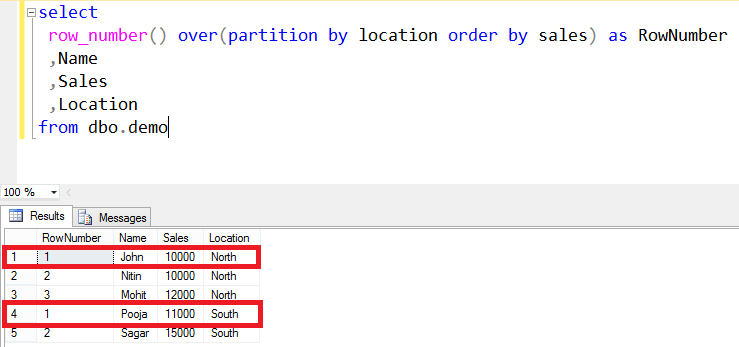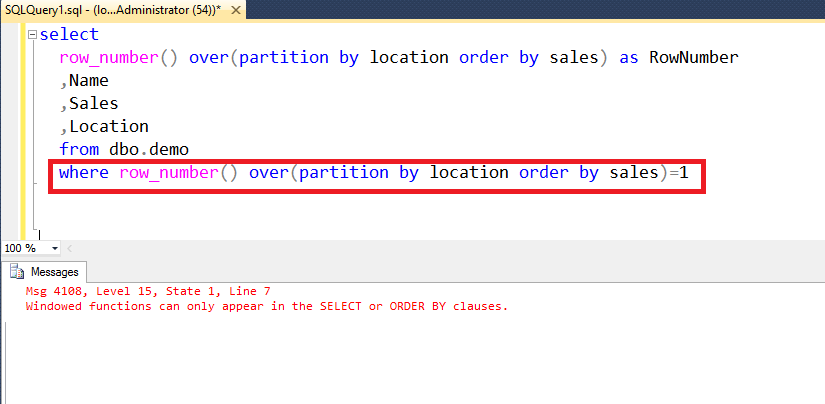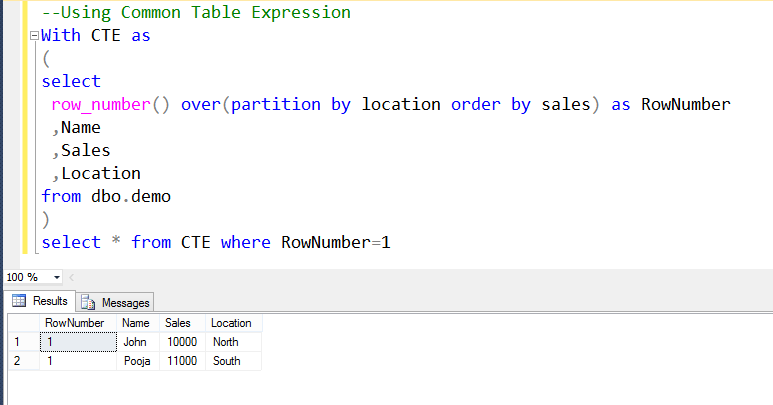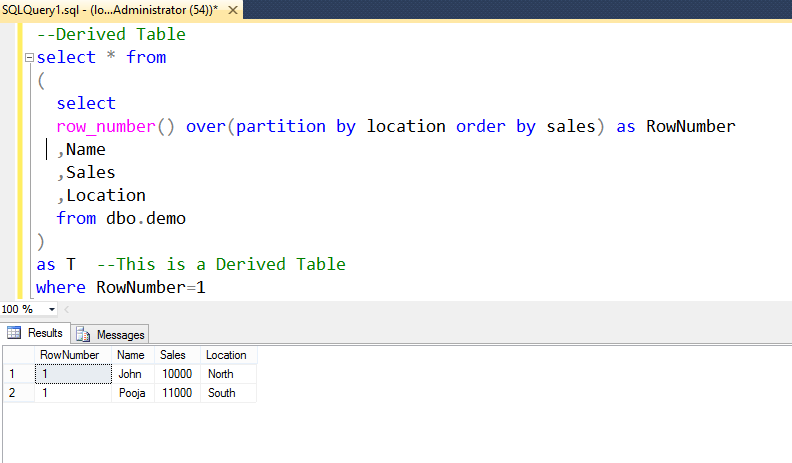# In this post i will discuss how to use row_number function in where clause in SQL Server.This post is also valid for other window functions like rank(),dense_rank(),ntile() etc.

Let us consider the T-SQL query which is using a window function named row_number().
The data was also partitioned by two locations North and South.
I am interested in only first record of the North location and first record of the South location
highlighted in the image below.When I tried to use row_number function in where clause I got the error number 4108 saying
Windowed functions can only appear in the SELECT or ORDER BY clauses.” This means that Window functions can’t be used in the where condition in T-SQL as shown in the image below.Now I am writing two solutions of this problem.

1. One can use row_number function in where clause in SQL server by Common Table Expression (CTE) as shown in the image below.I put the complete command in the Common Table Expression which created a temporary result set and later i referred that result set in the Select command and applied the filter condition.

2 ) I prefer CTE (Common Table Expression ) method over Derived Table as CTE is easier to read and understand.

But i am also providing Derived Table method as shown below.In the image above i put the whole query (which was using row_number function) as a subquery that is used in the FROM clause of the SELECT statement .Then in the outer SELECT statement i used the filter condition.